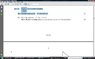# Matrices and quadratic basics help

Taylor_1989
I have figured out the answer to the question, but I have no idea why and how it works.

I have attached a copy of the question. I do apologize I am still having trouble putting into latex, I can install some but not all, so bare with me.

So if I multiple out the matrices I get $\chi$2 + 10$\rightarrow$ I then minus this from the quadratic $\chi$2 + 8$\chi$ + 10 = 0 $\rightarrow$ Which then gives me 8$\chi$ = 0

reagrange and I have $\chi$ = -8

Which is the right answer, I checked the mark scheme but I am suppose to find the value of K and not x. This make me think I have done the wrong maths but got the right answer.

Could someone point out if I have gone wrong, it would be very helpful.

It is the one highlighted.

#### Attachments

•matrices.jpg
27 KB · Views: 414

Hey Taylor_1989 and welcome to the forums.

Expanding your equation gives x^2 + 10 = kx which implies x^2 - kx + 10 = 0. But we know the equation is x^2 + 8x + 10 = 0 which means -k = 8 so k = -8.

Remember you know the equation, and you are finding the value of k when you expand your matrix multiplication and collect terms: solving for x is finding the roots of the function where you are solving x^2 + 8x + 10 = 0 for the variable x.

Taylor_1989
Hey Taylor_1989 and welcome to the forums.

Expanding your equation gives x^2 + 10 = kx which implies x^2 - kx + 10 = 0. But we know the equation is x^2 + 8x + 10 = 0 which means -k = 8 so k = -8.

Remember you know the equation, and you are finding the value of k when you expand your matrix multiplication and collect terms: solving for x is finding the roots of the function where you are solving x^2 + 8x + 10 = 0 for the variable x.

Thanks for the help, for some reason I got my equations mixed up, it nevered occurred to me to put Kx into a quadratic and then compare. I should have spotted it really. Well learn by your mistakes. Once again many thanks